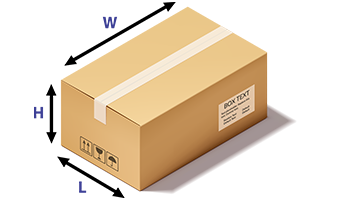AnexVM

In the logistics and cargo industry, the shipments are charged based on the actual weight or the volumetric weight (also called as dimensional weight). The customer is charged by transporter based on the weight, whichever is higher. The actual weight of the box can be easily measured by common electronic weighing machines, but there are very few machines that accurately calculate the volumetric weight of a parcel.

### What is Volumetric Weight of a parcel?

Volumetric or dimensional weight is the weight of the parcel which is calculated from its volume or dimensions. It is the space occupied by the box in terms of its density. For shipments, whose weight is less but is large in size, the dimensional weight is considered for costing. It is calculated to know to the space the shipment occupies on the pallet or the vehicle.### How to calculate volumetric weight of a parcel.

• The logistics and shipping industry basis its prices majorly on the actual weight of the package or the volumetric/dimensional weight of the box. The actual weight of the box can be easily calculated by placing it on a weighing scale and there are many instruments available in the market. The calculation for the volumetric weight of a package requires its exact dimensions and a formula to convert it to a weight equivalent...

#### For air shipments

The volumetric weight of the shipment is calculated by dividing the volume of the box by a factor 5000.

For example, consider a box of dimensions 50cm x 50cm x 50cm and the actual weight as 18 kgs.

= 50 x 50 x 50

5000

= 1,25,000

5000

Volumetric weight = 25 Kg

The shipment will be charged at 25kgs and not 18kgs

The volumetric weight of the shipment is calculated by dividing the volume of the box by a factor 6000.

For example,consider a box of dimensions 50cm x 50cm x 50cm and the actual weight as 18 kgs.

= 50 x 50 x 50

6000

= 1,25,000

6000

Volumetric weight = 21 Kg

The shipment will be charged at 21kgs and not 18kgs

#### Calculating volumetric weight saves cost.

• Most volumetric calculations are done manually. This is also done by deciding an approximate value for the dimensions and calculating the volumetric weight of the box.

• Consider an example, the size of the box is 55cm x 52cm x 50 cm and the actual weight of the box is 18 kgs. The volumetric weight is 28.6kgs.

• The cost of shipping this box from Bangalore to Delhi, considering Rs. 80/kg would be 1440 as per the actual weight. But by volumetric weight it would cost 2288, which is a huge difference of Rs. 848 for this one box shipment.

• If there are 100 boxes being shipped per day, that would sum to losses of 848x100 which is Rs. 84800 for a day for the shipper.